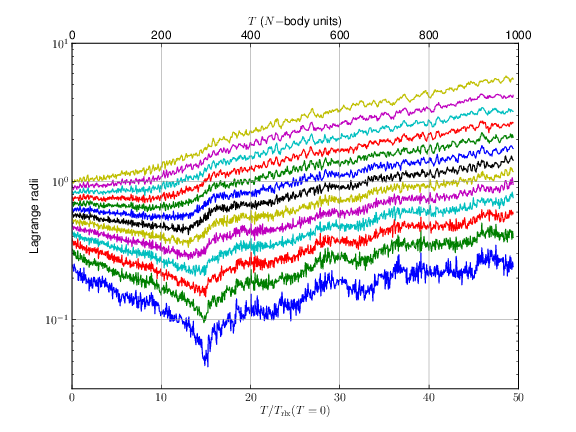# Preliminary results¶

We performed a set of experiments and we presented them into the “Star Clusters and Black Holes in Galaxies Across Cosmic Time” (IAU Symposium #312) under the title GraviDy, a modular, GPU-based, direct-summation N-BODY integrator, C. Maureira-Fredes & P. Amaro-Seoane.

## Experiments setup¶

The computational environment used for the following experiments was:

• CPU, Intel(R) Xeon(R) CPU X5650 @ 2.67GHz (24 cores)
• GPU, Tesla M2050 @ 575 Mhz (448 cores).
• RAM, 24 GB
• OS, Scientific Linux release 6.4

## Results¶

### Globular cluster evolution¶

Lagrange radii of an N- body system with 1024 particles, the lines in the plot shows the radii distribution, using 5%, 10%, 15%, ..., 65% of the total mass. The core collapse is reached at $$T_{\rm cc}\,\approx\,15\,T{\rm rh}_{t=0}$$, with a initial half-mass relaxation time of $$T{\rm rh}_{t=0}\,=\,20.24$$ NBU.Cumulative energy error up to $$t=1$$ NBU as a function of $$\eta$$. All the plots represent Plummer spheres with different amount of particles (N).Clock time up to $$t=1$$ NBU as a function of $$\eta$$. All the plots represent Plummer spheres with different amount of particles (N).### Performance¶

Clock time of integration from $$t=1$$ to $$t = 2$$ NBU using $$\eta = 0.01$$ and $$\epsilon = 0.0001$$ using different amount of particles (N).The following plot shows the acceleration of five different implementations using parallel computing techniques, compared to the single-thread base run.

• OpenMP, ...
• CPU + GPU, ...
• MPI-1, ...
• MPI-2, ...
• GPU, ...GPU gravitational interactions performance in GFLOPS for different amount of particles. The blue line at the top corresponds to the theoretical peak of the double precision floating point performance.